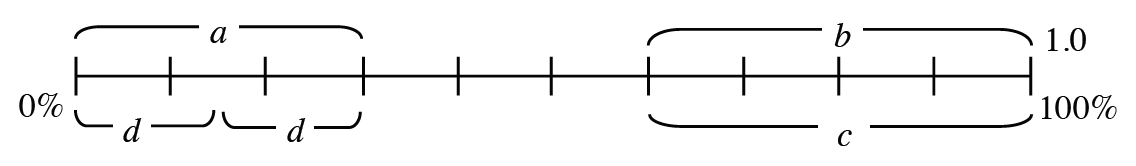### Home > MC2 > Chapter 4 > Lesson 4.3.3 > Problem4-100

4-100.

Find the missing lengths or values on the diagram below. Assume that the line is evenly divided.How many sections is this line divided into? Can you use this to find the value of each section?

Since the line is divided into $10$ sections, the sections each represent $10\%$ or $0.1$ of the whole.

To solve, note how many sections each bracket includes.

$a=0.3$
$b$ - Use the method above to solve.
$c=40\%$
$d$ - Use the method above to solve.
Note that the two sections are equal.